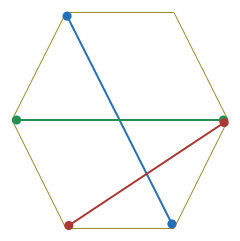Tricky hexagon!

Probability Level 3In a convex hexagon, two diagonals are drawn at random. What is the probability that the diagonals intersect inside the hexagon at 1 point?

×